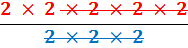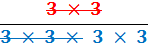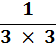### Dividing Powers

When you divide two powers with the SAME base number, you subtract the indices.

#### Example 1.   work out 25/23

in this example the base is 2

 25 = 2 × 2 × 2 × 2 × 2 = 32 23 = 2 × 2 × 2 = 8

 25/23 === 25−3    =    22 = 4

#### Example 2.   work out 32/34

in this example the base is 3

 32 = 3 × 3 = 9 34 = 3 × 3 × 3 × 3 = 81

 32/34 ==== 32−4   =   3−2 = 1/32   =   1/9to: